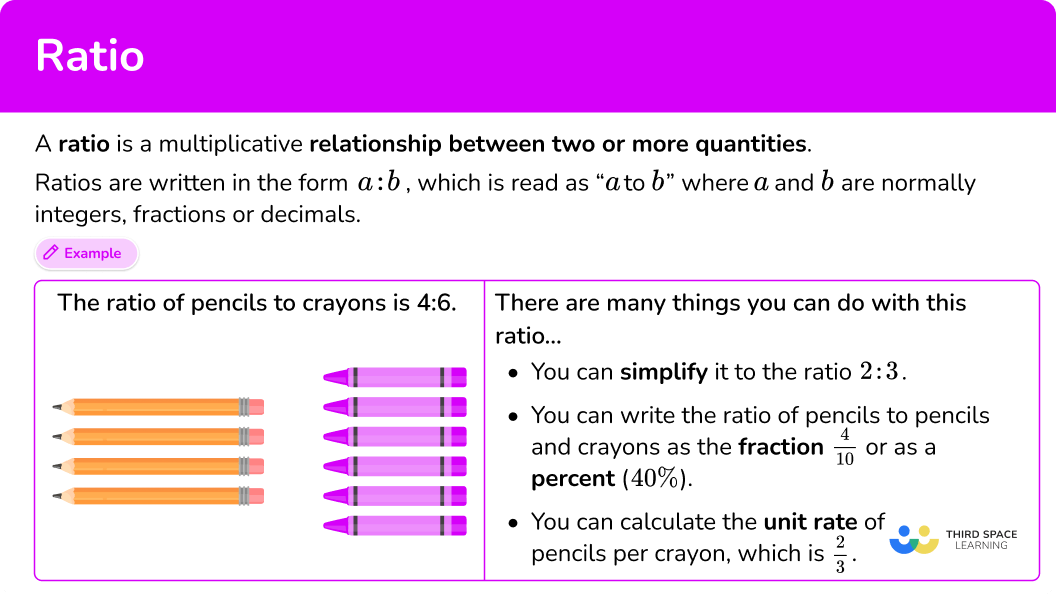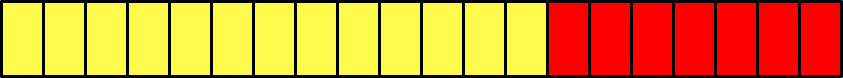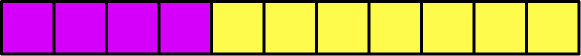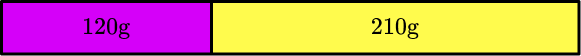# Ratio

Here you will learn about ratios, including how to write a ratio, simplifying ratios, unit rate math and how to solve problems involving ratios and rates.

Students will first learn about ratios as part of ratios and proportions in 6 th grade and 7th grade.

## What is a ratio?

A ratio is a multiplicative relationship between two or more quantities.

Ratios are written in the form a : b, which is read as “a to b”, where a and b are normally integers, fractions, or decimals.

The order of the quantities in the ratio is important.

For example,

If there are 10 boys in a class and 15 girls, the ratio of boys to girls is 10 : 15 which is read as “10 to 15.” This is an example of a part to part ratio. You could also say the ratio of total students to girls is 15 : 25. This is an example of a part to whole ratio.

Step-by-step guide: How to write a ratio

Since a ratio represents a relationship, there is always more than one way to show it.

This includes unit rate math – which creates equivalent ratios where one part of the ratio is 1.

You can use unit rates to compare different quantities.

For example,

A grocery store sells a bag of 6 bananas for \$2.34 and a bag of 4 bananas for \$ 1.44.

Which bag has the better unit price?

Unit price means the price per 1 unit. In this case, the units are bananas. Divide each ratio to find the price for 1 banana.The bag of 4 bananas is \$0.36 per banana, which is cheaper than the bag of 6 bananas which is \$ 0.39 per banana.

Step-by-step guide: Unit rate math

Unit rates are not the only types of equivalent ratios. When simplifying fractions, use the common factors to divide all the numbers in a ratio until they cannot be divided further to write the ratio in lowest terms.

For example,

The ratio of red counters to blue counters is 16 : 12.

You can simplify the ratio to lowest terms by finding the greatest common factor \textbf{(GCF)} of each of the numbers in the ratio.

Factors of 16 \text{:} \, 1, 2, 4, 8, 16

Factor of 12 \text{:} \, 1, 2, 3, 4, 6, 12

The greatest common factor is 4. To simplify the ratio, you divide both sides by 4.

Step-by-step guide: Simplifying ratios

Another way to write ratios is by using fraction notation.

Fraction notation can be used to show a part to whole ratio relationships.

For example,

The bar model below shows the ratio of blue : red as 3 : 2 (3 to 2). There are 3 blue blocks, 2 red blocks and 5 blocks in total.

This part to whole relationship allows us to make statements like…

• \cfrac{3}{5} of the blocks are blue

• \cfrac{2}{5} of the blocks are red

• \cfrac{5}{5} of the blocks are blue or red

The ratio of blue : red as 3 : 2 can also be shown as a part to part fraction…

For example,

The fractions show the ratio relationship BETWEEN the blue and red blocks. This allows us to make statements like…

• The number of blue blocks is \cfrac{3}{2} larger than red

• The number of red blocks is \cfrac{2}{3} the amount of blue

Step-by-step guide: Ratio to fraction

Ratios can also be written with percents.

For example,

The ratio of pencils to crayons is 4 : 6.

The ratio has 10 parts, so the fractions are

\cfrac{4}{10} : \cfrac{6}{10}.

The numerator represents the numbers of the ratio, which show how many pencils or crayons there are. The denominator represents the total number of pencils and crayons.

You may be able to recognize what the fractions are as percents or you may need to use long division to help convert your fractions.

\cfrac{4}{10}=40 \%, so 40 \% are pencils.

\cfrac{6}{10}=60 \%, so 60 \% are crayons.

Step-by-step guide: Ratio to percent

Solving problems with ratios is common in the real world. One place that this shows up is in calculating exchange rates. An exchange rate is the rate at which the money of one country can be exchanged for the money of another country.

Using a currency’s exchange rate you can convert between US dollars and foreign currencies.

For example,

To convert from US dollars (USD) to Japanese yen (JPY), you must multiply by the exchange rate.

For example,

So \$15 \; USD would be ¥2,134.35 \; JPY because, \$ 15 \; USD \times 142.29=¥ 2,134 .35 \; JPY.

Step-by-step guide: How to calculate exchange rates

All the skills above are examples of ratio problem solving. When solving problems with ratios, it is important to ask:

• What is the ratio involved?
• What order are the quantities in the ratio?
• What is the total amount / what is the part of the total amount known?
• What are you trying to calculate?

In the classroom, ratio problem solving often comes in the form of real world scenarios or word problems.

For example,

\cfrac{8}{10} students are right handed. What is the ratio of left handed students to right handed students? (2 : 8)

Step-by-step guide: Ratio problem solving

### What is a ratio?## Common Core State Standards

How does this relate to 6 th grade math and 7 th grade math?

• Grade 6 – Ratios and Proportions (6.RP.A.1)
Understand the concept of a ratio and use ratio language to describe a ratio relationship between two quantities. For example, “The ratio of wings to beaks in the bird house at the zoo was 2 : 1, because for every 2 wings there was 1 beak.” “For every vote candidate A received, candidate C received nearly three votes.”

• Grade 6 – Ratios and Proportions (6.RP.A.2)
Understand the concept of a unit rate \cfrac{a}{b} associated with a ratio a : b with b 0, and use rate language in the context of a ratio relationship.

For example, “This recipe has a ratio of 3 cups of flour to 4 cups of sugar, so there is \cfrac{3}{4} cup of flour for each cup of sugar.” “We paid \$75 for 15 hamburgers, which is a rate of \$ 5 per hamburger.”

• Grade 6 – Ratios and Proportions (6.RP.A.3)
Use ratio and rate reasoning to solve real-world and mathematical problems, for example, by reasoning about tables of equivalent ratios, tape diagrams, double number line diagrams, or equations.

• Grade 6 – Ratios and Proportions (6.RP.A.3b)
Solve unit rate problems including those involving unit pricing and constant speed. For example, if it took 7 hours to mow 4 lawns, then at that rate, how many lawns could be mowed in 35 hours? At what rate were lawns being mowed?

• Grade 7 – Ratios and Proportions (7.RP.A.1)
Compute unit rates associated with ratios of fractions, including ratios of lengths, areas and other quantities measured in like or different units.

For example, if a person walks \cfrac{1}{2} mile in each \cfrac{1}{4} hour, compute the unit rate as the complex fraction \cfrac{\cfrac{1}{2}}{\cfrac{1}{4}} miles per hour, equivalently 2 miles per hour.

## How to work with a ratio

There are a lot of ways to work with a ratio. For more specific step-by-step guides, check out the ratio pages linked in the “What are ratios?” section above or read through the examples below.

## Ratio examples

### Example 1: how to write a ratio

Write the ratio of apples to pears.

1. Identify the different quantities being compared and their order.

There are 5 pears and 2 apples.

The order of the ratio is apples to pears.

2Write the ratio using a colon.

Apples : Pears

\hspace{0.7cm} 5 : 2

3Check if the ratio can be simplified.

5 and 2 only have a common factor of 1, so this ratio is already in its lowest terms (simplest form).

### Example 2: unit rate calculation – decimal

A car travels 303 miles in 6 hours. If the car travels the same number of miles each hour, what is the miles per hour rate?

303 miles in 6 hours → 303 : 6.

The miles ‘per hour’ refers to 1 hour. Divide each side of the rate by 6, to create a rate for 1 hour.

The car travels 50.5 miles each hour.

### Example 3: simplifying ratios

Write the ratio 48 : 156 in lowest terms.

Factors of 48=1, 2, 3, 4, 6, 8, 12, 16, 24, 48

Factors of 156=1, 2, 3, 4, 6, 12, 13, 26, 39, 52, 78, 156

GCF(48,156)=12

4 : 13 is in lowest terms.

### Example 4: solving a problem involving ratio to percents

The ratio of adults to children in a park is 11 : 14.

One-fourth of the adults are women. What percent of the people in the park are men?

11+14=25. There are 25 parts in total. The denominator is 25.

11 : 14 becomes \cfrac{11}{25} : \cfrac{14}{25}.

\begin{aligned}& \cfrac{11}{25}=\cfrac{44}{100}=44 \% \\\\ & \cfrac{14}{25}=\cfrac{56}{100}=56 \%\end{aligned}

You now know that 44 \% of the people are adults.

One-fourth of the adults are women.

\cfrac{1}{4} of 44 \%=11 \%.

11 \% of the people in the park are women and therefore 44-11=33 \% of the people are men.

### Example 5: converting from KRW / USD

₩5,000 \; KRW is equal to \$3.85 \; USD. What is the exchange rate from \$ \; (USD) to ₩ \; (KRW)?

When calculating the currency exchange rate from ₩ \; (KRW), you want to know how many ₩ \; (KRW) are equal to \$1 \; (USD). This is the ratio of to \$, so set up the rate as \cfrac{₩ 5,000}{\$3.85}. In this case, the base currency is \$ \; (USD), so divide both parts by 3.85, rounding the ₩ \; KRW is to the nearest whole:

\cfrac{₩ 5,000 \div 3.85}{\$3.85 \div 3.85}=\cfrac{₩ 1,299}{\$ 1}.

The exchange rate from \\; (USD) to ₩ \; (KRW) is 1,299. ### Example 6: ratio problem solving – mixed numbers Fruit Salad Recipe: • 2 \cfrac{1}{2} cups of blueberries • 2 \cfrac{1}{5} cups of orange slices • 1 \cfrac{1}{4} cups of strawberries • 2 cups of apple slices Write the ratio of the total cups of berries for every 1 cup of strawberries in the salad. There are 1 \cfrac{1}{4} cups of strawberries and 2 \cfrac{1}{2} cups of blueberries. You need to create the ratio of the total cups of berries (strawberries and blueberries) for every 1 cup of strawberries. First add 1 \cfrac{1}{4}+2 \cfrac{1}{2} to find the total cups of berries. \begin{aligned}& 1 \cfrac{1}{4}+2 \cfrac{1}{2} \\\\ & =\cfrac{5}{4}+\cfrac{5}{2} \\\\ & =\cfrac{5}{4}+\cfrac{10}{4} \\\\ & =\cfrac{15}{4}\end{aligned} Then write the ratio of total cups of berries to cups of strawberries. \cfrac{15}{4} : \cfrac{5}{4} Now multiply both sides of the ratio by \cfrac{4}{5}, to calculate the ratio of 1 cup of strawberries. \cfrac{15}{4} \times \cfrac{4}{5} : \cfrac{5}{4} \times \cfrac{4}{5} 3 : 1 There are 3 total cups of berries for every 1 cup of strawberries. *Note: To solve, you can also write the ratio \cfrac{15}{4} : \cfrac{5}{4} as the complex fraction \cfrac{\cfrac{15}{4}}{\cfrac{5}{4}} and find the quotient of the numerator and denominator. ### Teaching tips for ratio • There are many ways to engage students in ratios. One way is to introduce the golden ratio (based on Fibonacci’s sequence) and challenge students to look for it in the real world. Keep a chart or wall in the classroom for students to add any examples of this ratio that they find. • Incorporate as many examples of ratios in the classroom as you can – even across subjects. For example, have students write ratios about the “Word of the day” – from an English or Science class. Such as “Write the ratio of nouns to adjectives” or “Write the ratio of words with the letter ‘e’ to total words.” • As students work with ratios in different ways, keep track of successful solving strategies on a bulletin board or on chart paper. This allows students to see and utilize another students’ strategy, make connections between strategies and feel ownership in any ideas they help create. • Be mindful of how to progress with ratio topics. Typically whole number ratios are introduced first, then ratios with rational numbers. Ratios that involve compare only fractional (or decimal values), such as \cfrac{2}{3} : \cfrac{5}{6} or 0.45 : 0.34 are the most difficult for students. As always, be mindful of your state’s curriculum when making decisions on when to introduce certain ratio topics. ### Easy mistakes to make • Writing the ratio in the wrong order A common error is to write the parts of the ratio in the wrong order. For example, The number of dogs to cats is given as the ratio 12 : 13 but the solution is incorrectly written as 13 : 12. • Confusing ratios and fractions You can write a ratio with fraction notation. A part to whole fraction will have the same fractional language as a fraction. However, a part to part fraction will not. For example, The ratio of boys to girls is 2 : 3. Two ways to express this ratio are \cfrac{2}{3} or \cfrac{2}{5}. However, you must be careful how you read these fractions. You can say “ \cfrac{2}{5} of the kids are boys” but you cannot say “ \cfrac{2}{3} of the kids are boys.” Instead, say “The number of boys is \cfrac{2}{3} the number of girls.” • Not fully simplified A common error is to not find the greatest common factor when simplifying a ratio. For example, Simplify the ratio 12 : 18. Dividing both numbers by only 2 leaves a ratio of 6 : 9, which is not fully simplified. This can be simplified further by dividing by 3 to get the ratio 2 : 3, which is the correct answer. By dividing both numbers by the greatest common factor, 6, would get the ratio 2 : 3 in one step. ### Practice ratio questions 1. 500 people attended a concert. There were 240 boys. What is the ratio of boys to girls who went to the concert? 240 : 260260 : 240240 : 500500 : 240There are 500 people and 240 boys. 500-240=260. There are 260 girls. The order of the ratio is boys to girls. Boys : Girls 240 : 260 2. A musical requires 200 costumes. 140 costumes are for the background dancers. The rest are for the lead roles. Write the ratio of the costumes for lead roles to background dancers in the simplest form. 7 : 37 : 103 : 710 : 7There are 200 costumes. 140 costumes are for background dances. 200-140=60 lead role costumes The ratio order of the ratio is lead roles to background dancers Lead roles : Background dancers \hspace{1cm} 60 : 140 The greatest common factor of 60 and 140 is 20\text{:}3. A shop is selling the same pencils in two different packs. Which statement correctly compares the packs? Pack \textbf{A}\text{:} \; 5 pens cost \ 6.20

Pack \textbf{B}\text{:} \; 4 pens cost \$4.88 Pack A is \$ 1.32 cheaper per pencil than Pack B.Pack B is \$1.32 cheaper per pencil than Pack A.Pack A is \$ 0.02 cheaper per pencil than Pack B.Pack B is \$0.02 cheaper per pencil than Pack A.Offer A\text{:} \; 5 pencils cost \$ 6.20 → 5 : \$6.20Each pencil in Pack A costs \$ 1.24.

Offer B\text{:} \; 4 pencils for \$4.88 → 4 : \$4.88Each pencil in Pack B costs \$1.22. \$ 1.24-\$1.22=\$ 0.02.

Offer B costs \$0.02 cheaper than Offer A. 4. The fraction of bananas in a bowl is \cfrac{13}{20}. Calculate the ratio of bananas to other pieces of fruit in the bowl. 13 : 2013 : 713 : 337 : 20The total number of pieces of fruit is 20. The number of bananas is 13. As a bar model, this looks likeThe number of other pieces of fruit is therefore 7 (this is calculated by 20-13=7 or counting the number of red bars above). The ratio of bananas to other pieces of fruit is therefore 13 : 7. 5. Given the exchange rate between US dollars (USD) and New Zealand dollars (NZD) is \$ 1 \; USD=\$1.63 \; NZD, convert \$ 50 \; USD to New Zealand dollars (NZD). Round to the nearest cent.

\$81.50 \, NZD\$ 30.67 \, NZD\$67.32 \, NZD\$ 84.56 \, NZD\$50 \, USD=\$ \rule{0.5cm}{0.15mm} \, NZD

Since each US dollar is equal to \$1.63 \, NZD, multiply the USD by 1.63 to find the number of \$ \, (NZD).\$50 \, USD=\$ 81.50 \, NZD

6. A soap is made by combining lavender soap with lemon soap. Each bar of soap weighs 330 \, g. If the ratio of lavender to lemon is 4 : 7,   how many grams of lemon soap are in each bar?

110 \, g120 \, g188.6 \, g210 \, gAs there are 7+4=11   shares within the ratio330 \div 11=30 \, g   per shareThe amount of Lemon in the soap is equal to 7 \times 30=210 \, g## Ratio FAQs

What does ratio mean in math?

While the term ratio is used in a variety of ways in the real world, the definition of ratio in math is the comparison of two or more values that have a constant relationship. Some examples of ratios are “ 2 dogs to 5 cats” or “ 24 miles per hour.”

Are ‘ratio’ and ‘rate’ synonyms?

A rate is a special type of ratio that compares different units. They are not synonyms, since not all ratios are rates. However, all rates are ratios, so they can be called by either name.

How are ratios used in upper level mathematics?

Ratio understanding is expanded to include more complex comparisons that involve exponents, variables and/or polynomials. This extends to include ratio relationships in proportions and linear equations. As students progress in their learning, they will become comfortable graphing, creating tables and equations that represent ratio relationships.

## Still stuck?

At Third Space Learning, we specialize in helping teachers and school leaders to provide personalized math support for more of their students through high-quality, online one-on-one math tutoring delivered by subject experts.

Each week, our tutors support thousands of students who are at risk of not meeting their grade-level expectations, and help accelerate their progress and boost their confidence.